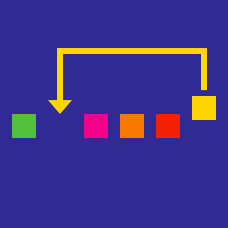Computer Science

# Binary Search

Consider the incomplete python implementation of the binary search algorithm:

  1 2 3 4 5 6 7 8 9 10 def BS( A , lo , hi, target ): if hi < lo: return -1 mid = (lo + hi)/2 if A[mid] == target: return mid elif A[mid] > target: return BS(A, MISSING_VARIABLE1 , MISSING_VARIABLE2, target ) #! else: return BS(A, MISSING_VARIABLE3, MISSING_VARIABLE4,target) #! 

By which variable names should MISSING_VARIABLE1,MISSING_VARIABLE2,MISSING_VARIABLE3,MISSING_VARIABLE4 be replaced respectively?

Suppose we want to find the root of the function $f(x) = k$, where $k$ is a positive real number. For which $f$ is a binary search applicable?

Which of the following is false about the binary search algorithm?

×